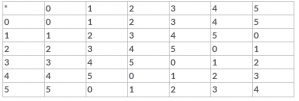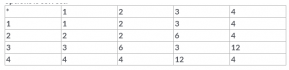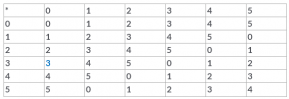Courses

# Test: Binary Operations

## 10 Questions MCQ Test Mathematics (Maths) Class 12 | Test: Binary Operations

Description
This mock test of Test: Binary Operations for JEE helps you for every JEE entrance exam. This contains 10 Multiple Choice Questions for JEE Test: Binary Operations (mcq) to study with solutions a complete question bank. The solved questions answers in this Test: Binary Operations quiz give you a good mix of easy questions and tough questions. JEE students definitely take this Test: Binary Operations exercise for a better result in the exam. You can find other Test: Binary Operations extra questions, long questions & short questions for JEE on EduRev as well by searching above.
QUESTION: 1

Solution:
QUESTION: 2

Solution:
QUESTION: 3

### Given below is the table corresponding to some binary operation a * b on a set {0,1,2,3,4,5}. How many elements of this operation have an inverse?.Solution:
QUESTION: 4

If * and O are two binary operations defined by a * b = a + b and a O b = ab, then​

Solution:
QUESTION: 5

The law a + b = b + a is called

Solution:
QUESTION: 6

The number of binary operations which can be defined on the set P= {p, q} is

Solution:
QUESTION: 7

Given below is binary composition table a* b = LCM of a and b on S = {1,2,3,4}. Then, from the table determine which one of these options is correct.Solution:
QUESTION: 8

Let * be any binary operation on the set R defined by a * b = a + b – ab, then the binary operation * is​

Solution:
QUESTION: 9

Given below is the table corresponding to some binary operation a * b on a set {0, 1,2,3,4,5}. Identify the identity element of this operation.Solution:
QUESTION: 10

The identity element for the binary operation * defined by a * b = ab/2, where a, b are the elements of a set of non-zero rational numbers, is​

Solution: